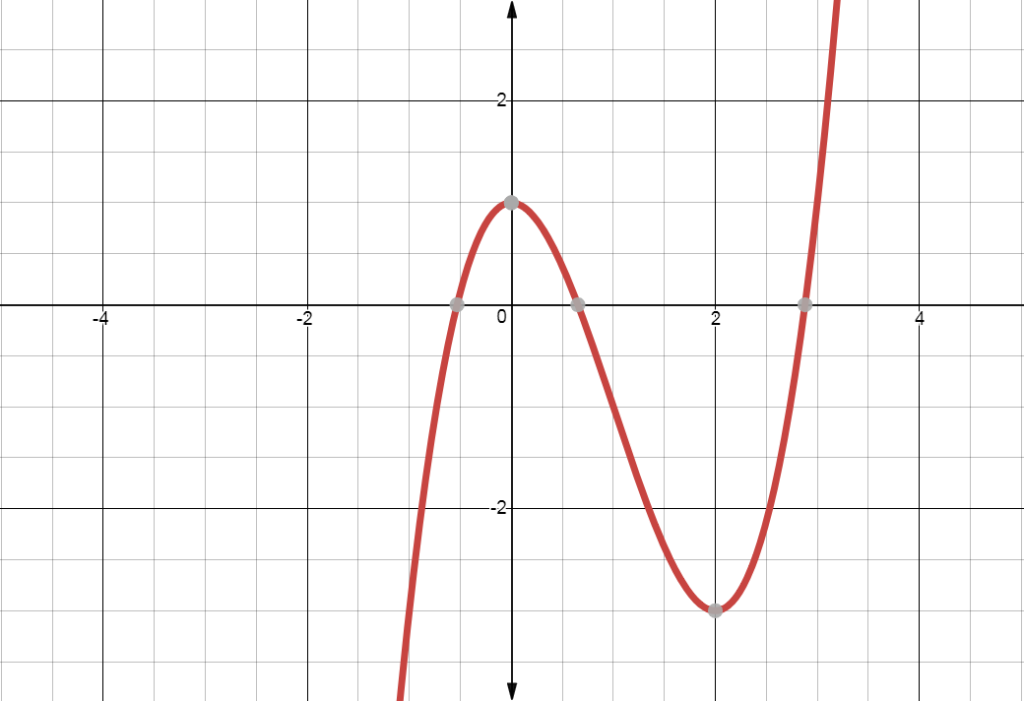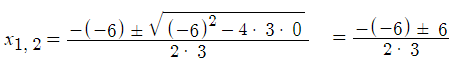# Local Maximum (Relative Maximum)

## What is a Local Maximum?

The local maximum (also called the relative maximum) is the largest value of a function, given a certain range. In other words, it isn’t the highest point on the whole function (that would be the global maximum), but rather a small part of it.

## Finding the Local maximum

Almost all functions have ups and downs. These are places where they can have a minimal or a maximal value. Of course, they have a lot of ups and downs, and we can’t find all of them at once. But, if we select a part of a function, then we can find the biggest and smallest value of that interval. This can be done by differentiating the function. For now, we’ll focus on the local maximum.

Let’s take this function as an example:

f(x) = x3 – 3x2 + 1If you consider the interval [-2, 2], this function has only one local maximum at x = 0. Here is how we can find it.

Step 1: Take the first derivative of the function f(x) = x3 – 3x2 + 1.

For this particular function, use the power rule. Place the exponent in front of “x” and then subtract 1 from the exponent. For example, d/dx x3 = 3x(3 – 1) = 3x2.

You will get a new function f′(x) which will look like this:

f′(x) = 3x2 – 6x

Step 2: Find the inputs where f′(x) is equal to zero. This means that you take the equation that you got in Step 1, and set it to equal zero.

3x2 – 6x = 0

From here on, use algebra to solve. This equation can be solved with the quadratic formula:

1.2.You should be able to see on the graph that the highest point of the function in x = 0. The other value x = 2 will be the local minimum of the function.

## Second Derivative Test

For the example above, it’s fairly easy to visualize the local maximum. But, there is another way to find it. If the function f′(x) can be derived again (i.e. by taking the second derivative), you can get to it by doing just that.

f′′(x) = 6x – 6

Now, we can replace the values that we got earlier (x = 0 and x = 2) in this function.

x = 0:

f′′(0) = 6 * 0 – 6

f′′(0)= – 6

A negative result (-6) means that x = 0 is the local maximum of the function. If the second derivative f′′(x) were positive, then it would be the local minimum.

x = 2:

f′′(2) = 6(2) – 6

f′′(2) = 12 – 6 = 6.

The solution, 6, is positive, which means that x = 2 is a local minimum.

## References

Stewart, J. (2009) Calculus: Concepts and Contexts. Cengage Learning.

CITE THIS AS:
Stephanie Glen. "Local Maximum (Relative Maximum)" From StatisticsHowTo.com: Elementary Statistics for the rest of us! https://www.statisticshowto.com/local-maximum/